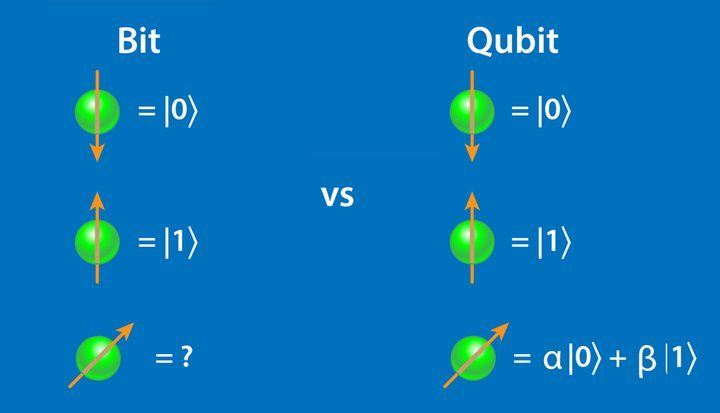# blog posts# Quantum computers are basically based on quantum physics and have a much higher computing power than supercomputers.

When it comes to quantum computers, many people probably think that we have a computer in the shape of current computers that has a much higher processing power.

This is a misconception and what we are talking about is basically supercomputers. The superpowers that have been competing for their development for years.

Quantum computers, however, are a completely different matter. They are basically based on quantum physics and have a much higher computing power than supercomputers.

For example, in late September of last year, we saw the introduction of Google’s first quantum computer called Sycamore with 53 qubits, which is extremely fast compared to the most powerful supercomputer in the world, Summit.

In other words, this quantum computer is able to perform calculations in 3 minutes and 20 seconds, which Summit needs 10,000 years to do.

## What is a quantum computer and how does it work?

As mentioned, the structure of quantum computers is different from that of classical computers. Classical computers work with transistors, and data is practically stored and used in bits of 0 and 1. As you know, bits definitely have a unit value of 0 or 1 at any given moment.

In quantum computers, however, instead of a transistor-based structure, they use manipulation of subatomic particles and spin (Spin is a property of subatomic particles that is a quantum property).

In these computers, instead of bits, we have quantum bits, which we call Qubit. We denote the base state of the qubits by | 0> and | 1> (and read as coat 0 and coat 1).

####Compare bits and qubits –

To better understand this, suppose we toss a coin, the moment the coin is fixed on the ground, it is definitely the shape of a coin. Now imagine that we roll the same coin on the edge, on the table. Can we say that a lion has appeared or a line while the coin is spinning? Definitely not.

In fact, at any given moment, the coin has a lion probability and a line probability at the same time.

The function of the qubits is basically the same as that of a coin circulating on the edge. Qubits use the Superposition property, which can have values ​​of 0 and 1 at the same time as a probability.

We show this with the following format and formula. The two numbers α and β are actually two complex numbers that apply to the following formula.

This superposition feature and other features such as entanglement make quantum computers ideal systems for certain computations.

## Is security compromised by quantum computers?

The subject of quantum computers has been discussed since the 1980s and will be used in the design and testing of new drugs, weather forecasting, data analysis, artificial intelligence, and more. But one of the applications of quantum computers in the field of computers is in the field of cryptography. As you know, the issue of security is quite relative, and what we call security today may not have any security in a few years.

Currently, the RSA algorithm is used as a secure algorithm on the Internet, in most communications that require high security, such as banking communications. The basis of this algorithm is the existence of a very large natural number such as N, which cannot be broken by the first factors (or power prime).

Finding these first factors of a large number is a very difficult task (NP-Complete) that takes billions of years to obtain on ordinary computers and even supercomputers. But quantum computers, in a very short time, due to their structure and high speed, can obtain these factors using algorithms such as Shor (which is one of the most famous and important quantum algorithms).

#### So the current security we have is generally in question.

However, this concern is not really present at this time because, although we have had quantum algorithms for many years, quantum computers are not available to us.

On the other hand, quantum computing itself can also help increase security. In fact, if we have a quantum communication channel, we can transfer data and keys through this secure channel and experience unparalleled security.

Some work has already been done on quantum computers. Examples include IBM and Google quantum computers, as well as Honeywell’s new 10-qubit H1 quantum computer .

Finally, quantum computers can be used to solve problems that require a lot of memory and computing, but it seems that the use of supercomputers in the future will be even more than quantum computers.